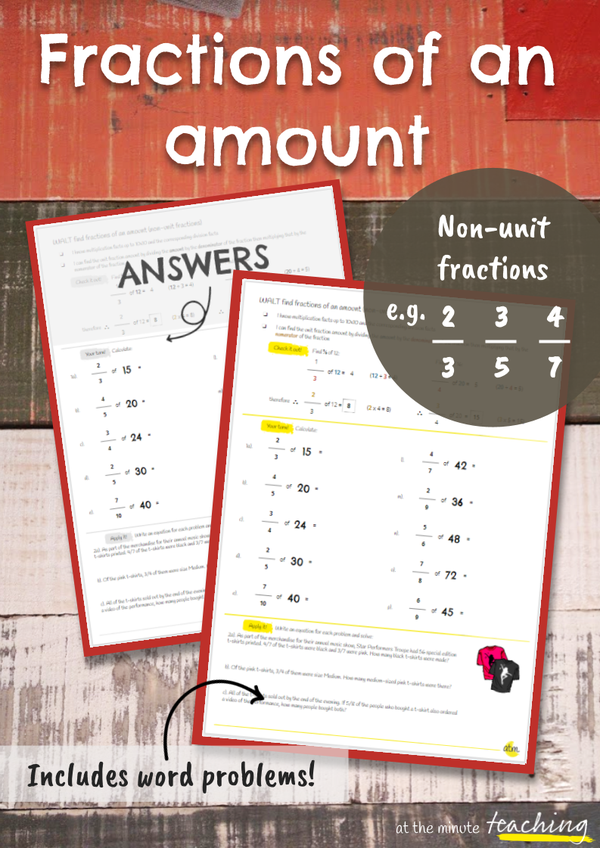#### attheminute teaching

Be purposeful teachers
Who are in control
Feel inspired
And know we've done enough.Kath
Teaching/Shop/Maths/Fractions of an amount - Non-unit fractions worksheet

# Fractions of an amount - Non-unit fractions worksheet## R 10,00ZAR

#### Description

In this worksheet, students will calculate fractions of an amount by dividing by the denominator and multiplying by the numerator. Students need to know multiplication and corresponding division facts up to 10x10.

• Maths

#### Details

In this worksheet, students will calculate fractions of an amount by dividing by the denominator and multiplying by the numerator. Students need to know multiplication and corresponding division facts up to 10x10.

A WALT and success criteria/key mathematical knowledge are included at the top of the worksheet. There are 10 practice questions and 3 word problems which students need to write out a matching equation for before solving.

Answers are included as well as grayscale versions of the question and answer sheets.

Need to go back a step? Grab 'Fractions of an Amount - Unit Fractions' here.

### Other resources you might like:

#### attheminute teaching

Be purposeful teachers
Who are in control
Feel inspired
And know we've done enough.Kath